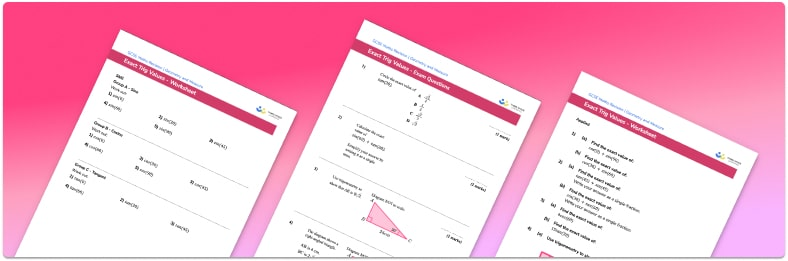# Exact Trig Values Worksheet• Section 1 of the exact trig values worksheet contains 14 skills-based exact trig values questions, in 3 groups to support differentiation
• Section 2 contains 4 applied exact trig values questions with a mix of worded problems and deeper problem solving questions
• Section 3 contains 4 foundation and higher GCSE exam style questions on exact trig values
• Answers and a mark scheme for all exact trig values questions are provided
• Questions follow variation theory with plenty of opportunities for students to work independently at their own level
• All questions created by fully qualified expert secondary maths teachers
• Suitable for GCSE maths revision for AQA, OCR and Edexcel exam boards
• Interactive lessons and worksheets are available for a wide range of geometry and measure topics

• This field is for validation purposes and should be left unchanged.

You can unsubscribe at any time (each email we send will contain an easy way to unsubscribe). To find out more about how we use your data, see our privacy policy.

### Exact trig values at a glance

The sin, cos and tan of certain angles are exact values rather than decimals. The exact trigonometric values of sin, cos and tan are:Knowing the exact values of sin, cos and tan allows us to use the trigonometric functions without using a calculator for certain angles.

These values can be found by applying the trigonometric ratios of sin, cos and tan to two special triangles:For example, using the trig ratio sin(x) = opposite/hypotenuse we get sin(45) = 1/square root of 2.

Applying each of the trig functions to these triangles will give the exact trig values for 30o, 45o and 600

Looking forward, students can then progress to additional geometry worksheets, for example an orFor more teaching and learning support on Geometry our GCSE maths lessons provide step by step support for all GCSE maths concepts.

## Do you have KS4 students who need more focused attention to succeed at GCSE?There will be students in your class who require individual attention to help them succeed in their maths GCSEs. In a class of 30, it’s not always easy to provide.

Help your students feel confident with exam-style questions and the strategies they’ll need to answer them correctly with our dedicated GCSE maths revision programme.

Lessons are selected to provide support where each student needs it most, and specially-trained GCSE maths tutors adapt the pitch and pace of each lesson. This ensures a personalised revision programme that raises grades and boosts confidence.

Find out more